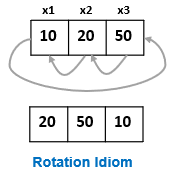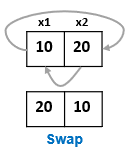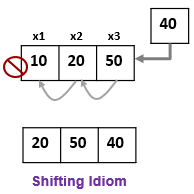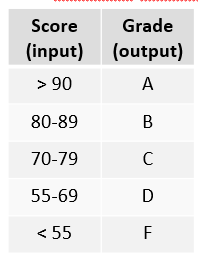# CSCI 102 - Spring 2020 Fundamentals of Computation

• Integer Division & Modulo Idioms
• Assignment Idioms
• Conditional Idioms
• Loop Idioms

## Integer Division / Modulo

### Unit Conversion Idiom

• Name : Unit conversion
• Motivating Example(s):
• Cents to change (e.g. quarters, dimes, ...)
• Ounces to pounds, Items to Dozens, Feet to miles, etc.
• Description : Convert a single value into larger units.
• Structure: To convert a number n in units-A to units-B (where the conversion factor is k units-A per unit-B): use the integer division, n / k, to determine the integral (whole) number of units-B and n % k to determine the remaining (leftover) units-A.

• Example(s):

• Convert the given number of ounces to pounds (lbs.) and pounds. There are 16 ounces per pound.
    int oz, lbs;
cin >> oz; // get the number of ounces
cout << oz << " ounces => ";

lbs = oz / 16;  // Convert to the number of pounds
oz = oz % 16;   // Update remaining ounces
cout << lbs << " pounds, " <<  oz << " ounces." << endl;


### Isolating Digits Idiom

• Name : Isolating Digits

• Description : Extract the k least-significant digit(s) of a number n (e.g. given n=1234, extract the 1's digit, 4 or the last 2 digits, 34, etc.). You can also extract the more-significant digits (i.e. the digits above the k least-significant digits).

• Structure: The least significant digits can be accomplished with the modulo (%) operator by taking the remainder of n % 10^k (i.e. divide n by 10 to the power of k and take the remainder). Finding the more significant digits beyond the lower k digits can be found by simply using integer division of the same two values: n / 10^k.

• Example(s):

• Find the 1's digit of a number
    int n;
cin >> n; // get an integer from the user

cout << "The 1s digit of " << n << " is " << n % 10 << endl;

• To determine the level of a USC class number (1xx, 2xx, 3xx, 4xx, 5xx, etc.)
    int n;
cin >> n; // get an integer like 102, 155, etc.

cout << "Your class' level is " << n/100 << endl;


### Divisibility/Factor Idiom

• Name : Divisibility/Factor Check
• Description : Check whether a number, n, is divisible by some other number, k, (i.e. k is a factor of n) by checking if the remainder of n divided by k is 0.
• Structure: This check can be accomplished with the modulo (%) operator and checking if n % k yields 0.
• Example(s):

• Check if a user provided number, n, is divisible by 3
    int n;
cin >> n; // get an integer from the user

if(n % 3 == 0){
cout << n << " is divisible by 3." << endl;
}

• To check if a number is even or odd, simply check if a number is divisible by 2.
    int n;
cin >> n; // get an integer from the user

if(n % 2 == 0){
cout << n << " is even" << endl;
}
else {
cout << n << " is odd" << endl;
}


## Assignment Idioms

### Swap (Rotation)

• Name: Swap
• Motivating Example(s): Swap or exchange the value of two (or potentially more) variables
• Description: Use a temporary variable (to hold a copy of one of the variables) and three assignment statements.
• Related to: Moving Window Idiom
• Structure: Use a temporary variable (to hold a copy of one of the variables) and three assignment statements. Start by making a copy of the one of the variables and then each successive assignment should overwrite the variable that was just copied.// variables to be swapped
int x1, x2;

int temp;

// Shift each item in reverse order
// overwriting the value to be dropped first
temp = x1;      // Make a copy of the first variable
x1 = x2;        // Now overwrite the first with the second
x2 = temp;      // Replace the second with the copy of the first

• Example(s):

• Given two integers, a and b, put the greater number into a and the smaller in b
c++ int a, b; cin >> a >> b; // Get two numbers

// Check if b is greater than a
if(b > a) {
// Swap the value so the greater values is in a
int temp = a;
a = b;
b = temp;
}

// Otherwise the larger number is already in a


### Moving-Window (Shifting Values)

• Name: Moving-window
• Motivating Example(s): Track the best or newest n values,
• Description: Use k variables to store values such that when one is added, other values shift variable locations and one value is dropped. In it's simplest form (k=1) we only need to store 1 variable and overwrite it each time we get a new value, but it becomes more error-prone when k > 1.
• Related to: Swap Idiom
• Structure: Carefully order assignment statements such that you overwrite (assign) the variable to be dropped (oldest) first and then move each value in "reverse" order until you reach the variable that should get the newest value, at which point you assign the new value to that variable. Be careful not to go in the wrong order (newest to oldest) or you will lose all your values.// k variables
//  assume x1 holds the best/newest value while
//         xk holds the worst/oldest value
int x1, x2, ...,  xkm1, xk;

int new_value;
cin >> new_value; // get a new value

// Shift each item in reverse order
// overwriting the value to be dropped first
xk = xkm1;      // Replace the oldest
...             // with 2nd oldest
x3 = x2;
x1 = new_value; // The newest is now update with the latest value

// We can repeat and do it again
cin >> new_value; // get another new value

// Repeat the same assignments as above
xk = xkm1;
...

• Example(s):

• Produce the sum of the last two numbers entered by the user (e.g. if the user enters: 4 6 2 7 5 produce the output: 10 8 9 12).

    int last, current;
cin >> last >> current;  // Get the first two numbers to get started
cout << "Last 2 numbers sum to: " << last + current << endl;

// Now we'll get one new number at a time, but before we overwrite
// current we shift it to become the last value.
last = current;
cin >> current;
cout << "Last 2 numbers sum to: " << last + current << endl;

last = current;
cin >> current;
cout << "Last 2 numbers sum to: " << last + current << endl;

last = current;
cin >> current;
cout << "Last 2 numbers sum to: " << last + current << endl;

• A certain wealthy businesswoman has a 3 car garage. Because there is only room for 3 cars, each time she purchases a new car she has to sell the car she's had the longest. The program below tracks which cars are in her garage at each point in time

    string c1, c2, c3; // c1 is the newest car and c3 the oldest
cin >> c3 >> c2 >> c1;  // She can store her first 3 cars
// Assume user types in make/model (e.g. "Tesla Model 3")

cout << "Owned cars: " << c1 << " " << c2 << " " << c3 << endl;

// Now she'll get a new car but needs to sell her oldest
c3 = c2;    // 2nd oldest becomes the oldest (oldest is dropped)
cin >> c1;  // Input the make/model of the new car
cout << "Owned cars: " << c1 << " " << c2 << " " << c3 << endl;

c3 = c2;    // 2nd oldest becomes the oldest (oldest is dropped)
cin >> c1;  // Input the make/model of the new car
cout << "Owned cars: " << c1 << " " << c2 << " " << c3 << endl;

• Common Mistakes:

• Because statements execute sequentially (one after the next) and what we assign to a variable in one step is the value that will be used by the next statement to reference that variable, if we assign in the wrong order we will overwrite all our data as in the example below.

    string c1, c2, c3; // c1 is the newest car and c3 the oldest
cin >> c3 >> c2 >> c1;  // She can store her first 3 cars
// Assume user types in make/model (e.g. "Tesla Model 3")

cout << "Owned cars: " << c1 << " " << c2 << " " << c3 << endl;

//*************************************************************
// Do NOT use this code - it is an example of what *NOT* to do.
//*************************************************************

// Assigning in the wrong order will overwrite all our data
// Suppose c1 was "Tesla", c2 was "BMW" and c3 was "Audi"
cin >> c1;  // We now input "Lexus" overwriting "Tesla"
c2 = c1;    // We then take "Lexus" (new val of c1) and overwrite "BMW"
c3 = c2;    // And then take "Lexus" from c2 and overwrite "Audi"
cout << "Owned cars: " << c1 << " " << c2 << " " << c3 << endl;

// This would print: "Owned cars: Lexus Lexus Lexus"

• Exercise(s):

• Understand the code above for outputting the sum of the last two numbers the user enters and consider how you can extend it if we want to produce the sum of the last 3 numbers entered, rather than last 2.

## Conditional Idioms

### Lookup Table

• Name: Look-up table (Parallel cases)
• Motivating Example(s):
• Determine win, lose, or draw based on score
• Applying different rules based on age, year in school, or some other characteristic
• Description: Break input options into mutually exclusive cases (or ranges) and convert (aka map) each input option to another output option
• Structure: Use a single level (not nested) if...else if...else structure to check for each input case and convert to the desired output value.// check each case
if( /* Case 1 condition */ ) {
// Produce output 1
}
else if( /* Case 2 condition */ ) {
// Produce output 2
}
else if( /* Case 3 condition */ ) {
// Produce output 3
}
else {
// Produce default output
}

• Example(s):

int pct;
cin >> pct;  // Get user input

// Output all the odd numbers
if(pct >= 90){
cout << "A" << endl;
}
else if(pct >= 80){
cout << "B" << endl;
}
else if(pct >= 70){
cout << "C" << endl;
}
else if(pct >= 60){
cout << "D" << endl;
}
else {
cout << "F" << endl;
}

• Determine if a number if positive, negative, or 0

int n;
cin >> n;  // Get user input

// Output all the odd numbers
if(n > 0){
cout << "Positive" << endl;
}
else if(n < 0){
cout << "Negative" << endl;
}
else {
cout << "Zero" << endl;
}


### Decision Tree

• Name: Decision Tree (Sequential cases)
• Motivating Example(s):
• Help systems such as medical diagnosis, computer or automotive repair, etc.
• Multi-level menus (customer service or hierarchical organization)
• Description: Use a sequence of checks or process of elimination to identify a particular case and perform an appropriate action.
• Structure: Use nested if..else if...else statements. The outer if..else if statements check the most general cases (or the first level criteria) while the inner if...else if...else structure checks more specific values (or the next level criteria).// check each case
if( /* Top-level condition 1 */ ) {

// Nested if statement for Next-level condition
if( /* Next-level condition A */ ) {

}
else if ( /* Next-level condition B */ ) {

}
else { /* Next-level condition C */

}
}
else if( /* Top-level condition 2 */ ) {
// Nested if statement for Next-level condition

}
else {  /* Top-level condition 3 */
// Nested if statement for Next-level condition

}

• Example(s):

• Automated customer service
if( option1 == "accounts" )  {
// account related services
if( option2 == "balance" ) {
// Code to retrieve balance
}
else if(option2 == "cancel" ) {
// Code to cancel account
}
}
else if (option1 == "hours")
{
// code to list hours
}
else
{
// repeat options
}

• Choosing a major based on personal interest
    string general_interest, specific_interest;
cin >> general_interest >> specific_interest;

string suggested_major;
if(general_interest == "engineering"){
if(specific_interest == "software"){
suggested_major = "CSCI";
}
else if(specific_interest == "cars"){
suggested_major = "ME";
}
else {
suggested_major = "EE";
}
}
else if(general_interest == "sciences"){
if(specific_interest == "medicine"){
suggested_major = "BIO";
}
else if(specific_interest == "astronomy"){
suggested_major = "PHYS";
}
else {
suggested_major = "CHEM";
}

}
else if(general_interest == "humanities"){
if(specific_interest == "literature"){
suggested_major = "ENGL";
}
else {
suggested_major = "PSYC";
}
}
else {
}

• Notes: This can be implemented with one level of if/else statements using logical-AND operators (&&) where general conditions are repeated with each specific condition (e.g. if(general_interest == "engineering" && specific_interest == "software") and else if("general_interest == "engineering" && specific_interest == "cars")).

### Rule / Exception Idiom

• Name : Rule/Exception
• Description : Perform a default action and then us an if to correct for exceptional cases
• Structure: Code for some default action (i.e. the rule) is followed by if statement with code to correct the exceptional case

// Default action

if( /* Exceptional Case */ )
{
// Code for exceptional case
}

• Example(s):

• Base pay plus bonus for certain exceptional employees
    bool earnedBonus = /* set somehow */;
int bonus = /* set somehow */;

int basePay = 100;
if( earnedBonus == true )
{
basePay += bonus;
}

• Notes: This can be implemented with an if/else where an if implements the rule (or exception) and the else implements the other.

## Loop Idioms

### Map

• Name: Map
• Motivating Example(s):

• Map numeric scores to letter grades
• For each x compute f(x) (e.g. output the first n odd integers, n : 2*n+1)
• Description: Convert (map) each value in a collection to another value

• Related to: Often combined with the Selection idiom (if reducing only values that meet some criteria)

• Structure: Loop through each element and apply the desired operation.

// loop through each instance
for( /* each instance, i */ ) {
// Map each value based on i
}

• Example(s):

• Output the first n positive, odd numbers

int n;
cin >> n;  // Get user input

// Output all the odd numbers
for( int i = 0; i < n; i++ ) {
cout << 2*i + 1 << endl;
}

• Output the value of f(x) = ax^2 + bx + c for x in the range [-10,+10] with a stepsize of 0.2

double a, b, c, x;
cin >> a >> b >> c;  // Get the coefficients: a, b, c

// Generate each value of x
for( x = -10; x <= 10; x += 0.2 ) {
// Now map x to f(x)
cout << a*x*x + b*x + c << " ";
}
cout << endl;


### Reduce

• Name: Reduce / Combine / Aggregate
• Motivating Example(s):
• Compute the average of a collection of numbers
• Perform numerical integration of a function over a range
• Description: Combine/reduce all elements of a collection to a single (or constant number of) value(s)
• Related to: Often combined with the Selection idiom (if reducing only values that meet some criteria)
• Structure: Declare and initialize a "reduction" variable to store the combined/aggregated value (usually initalizing it to the identity function for the given operation such as 0 for addition, 1 for multiplication, etc.). Then loop through each element and combine the element with the "reduction" variable using the desired operation.

// reduction variable
int reduction_variable = identity_value; // (e.g. 0)
// loop through each instance
for( /* each instance, i */ ) {
reduction_variable = reduction_vaiable op i
}

• Example(s):

• Average the first n integers input by the user

int n, val;
cin >> n;  // The user first enters the number, n
// Then the user will enter n integers

int sum = 0; // Reduction variable
for( int i = 0; i < n; i++ ) {
cin >> val;
sum += val;
}
cout << "Average: " << (double) sum / n << endl;

• Integrate the value of f(x) = ax^2 + bx + c for x in the range [-10,+10] using dx=0.01

double a, b, c, x;
cin >> a >> b >> c;  // Get the coefficients: a, b, c

// Compute f(x) and integrate each small f(x)*dx area
double sum = 0.0, dx = 0.01;
for( x = -10; x <= 10; x += dx ) {
// Now map x to f(x)
sum += (a*x*x + b*x + c) * dx;
}
cout << endl;


### Selection

• Name: Selection
• Motivating Example(s):
• Find the minimum or maximum element from a collection
• Count how many students scored an A on the exam
• Description : Select a subset (possibly one or none) of elements from a collection based on a particular property
• Related to: Distributed-AND Idiom and Distributed-OR Idiom
• Structure: Loop through each element and check whether it meets the desired property. If so, perform a map, reduce, or other other update operation.

// declare/initialize any state variables needed
//  to track the desired result

// loop through each instance
for( /* each instance, i */ ) {
// Check if this instance meets the property
if(condition) {
// Update state (variables) appropriately
}
}
// The state variables now have the desired answer

• Example(s):

• Count how many students scored an A on the exam.
    int n, score;
cin >> n;  // User will enter how many scores they will enter

// declare/initialize any state variables needed
//  to track the desired result
int count = 0;
// loop through each instance
for( int i = 0; i < n; i++ ) {
cin >> score;
// Check if this instance meets the property
if(score >= 90) {
// Update state (variables) appropriately
count++;
}
}
// The state variables now have the desired answer
cout << count << " people got an A" << endl;


### Succeed Early / Fail At The End (Distributed-OR)

• Name : Distributed-OR
• Problem Characteristic: Succeed Early / Fail at the End
• Motivating Example(s):
• Check if anyone got a perfect score on an exam
• Search for a number in a collection
• Description : Determines if a property is true for SOME instance of a collection.
• Related to: Distributed-AND Idiom
• Structure: Loop through each instances and check whether an instance meets the desired property. If so, set a variable and stop the search. Only if we loop through all the instances can we prove no instance met the property, but if even just one case did meet the property we can stop.

    // default to false, then prove some instance meets
// the desired property
bool result = false;
// loop through each instance
for( /* each instance, i */ ) {
// Check if this instance meets the property
if(condition) {
// Set the result and stop
result = true;
break;
}
}
// if we made it through all iterations of the loop
// the property is false

• Example(s):

• Determine if any one got a perfect scores (i.e. 100) on an exam.
int n, val;
cin >> n;  // User will enter how many scores they will enter

bool result = false;
// loop through each case
for( int i = 0; i < n; i++ ) {
cin >> val;
// Check if this is a perfect score
if(val == 100) {
result = true;
break;  // Stop the search
}
}
// if we made it through all iterations of the loop
// the property is false
if(result == true) {
cout << "There is a perfect score" << endl;
}
else {
cout << "No perfect scores exist." << endl;
}

• Find the index in an array of a specific target value, or -1 if the target number does not exist. (Note: This example uses arrays which may or may not have been covered in your lecture.)
int data, target;
cin >> target;  // The user will enter the desired target
// to search for

int idx = -1;
// loop through each case
for( int i = 0; i < n; i++ ) {
if(data[i] == target) {
idx = i; // save the index where the target is located
break;   // Stop the search
}
}
// if we made it through all iterations of the loop
// the property is false
if(idx == -1) {
cout << target << " does NOT exist in the array" << endl;
}
else {
cout << target << " exists at index " << idx << endl;
}


### Fail Early / Succeed At The End (Distributed-AND)

• Name : Distributed-AND
• Problem Characteristic: Fail Early / Succeed at the End
• Motivating Example(s):
• Check if everyone passed the exam
• Determine if a number is prime
• Description : Determines if a property is true for ALL instances of a collection.
• Related to: Distributed-OR Idiom
• Structure: Loop through each case or condition. If it is false, we have proven the property doesn't hold for all instances and we quit the loop early (before checking the remaining instances. If it is true, move on to the next case. Not until we loop through all the instances can we prove the property is true, but if just one case is false we have proven the property is false and can stop.

// default to true, then prove it false
bool result = true;
// loop through each case
for( /* each instance, i */ ) {
if(condition) {
result = false;
break;
}
}
// check the result variable with an if statement

// if we made it through all iterations of the loop
// the property is true

• Example(s):

Check if all n numbers entered are 70 or more

int n, val;
cin >> n;  // User will enter how many values they will enter

// set a variable to true
bool result = true;
// loop through each case
for( int i = 0; i < n; i++ ) {
cin >> val;
if(val < 70) {
result = false;
break;
}
}
// if we made it through all iterations of the loop
// the property is true
if(result == true) {
cout << "All numbers are 70 or more" << endl;
}
else {
cout << "At least one number less than 70" << endl;
}
`